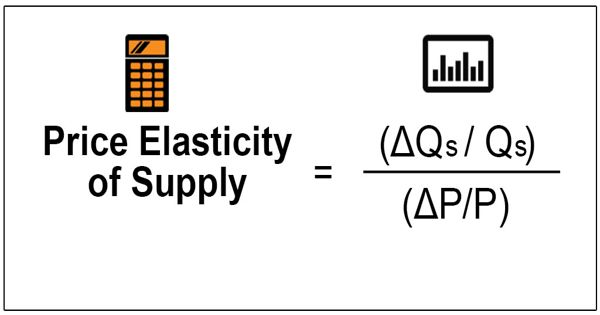Economics

# Price Elasticity of SupplyPrice elasticity measures the responsiveness of the quantity demanded or supplied of a good to a change in its price. The price elasticity of supply (PES or Es) is a measure used in economics to show the responsiveness, or elasticity, of the quantity supplied of a good or service to a change in its price. The concept of price elasticity of supply can be applied to labor to show how the quantity of labor supplied responds to changes in wages or salaries. It is the measure of the responsiveness in quantity supplied (QS) to a change in price for a specific good (% Change QS / % Change in Price).

Price elasticity of supply measures the responsiveness of quantity supplied to a change in price. It measures the responsiveness to the supply of a good or service after a change in its market price.

The price elasticity of supply is the percentage change in quantity supplied divided by the percentage change in price. The elasticity is represented in numerical form, and is defined as the percentage change in the quantity supplied divided by the percentage change in price. It is computed as the percentage change in quantity demanded—or supplied—divided by the percentage change in price.

The price elasticity of supply measures the responsiveness of quantity supplied to changes in price. When the elasticity is less than one, the supply of the good can be described as inelastic; when it is greater than one, the supply can be described as elastic. It is the percentage change in quantity supplied divided by the percentage change in price. There are numerous factors that directly impact the elasticity of supply for a good including stock, time period, availability of substitutes, and spare capacity. It is usually positive.

An elasticity of zero indicates that the quantity supplied does not respond to a price change: the good is “fixed” in supply. Supply is price inelastic if the price elasticity of supply is less than 1; it is unit price elastic if the price elasticity of supply is equal to 1, and it is price elastic if the price elasticity of supply is greater than 1. Such goods often have no labor component or are not produced, limiting the short-run prospects of expansion. A vertical supply curve is said to be perfectly inelastic. A horizontal supply curve is said to be perfectly elastic. If the elasticity is exactly one, the good is said to be unit-elastic.

The price elasticity of supply is greater when the length of time under consideration is longer because over time producers have more options for adjusting to the change in price. The number of goods supplied can, in the short term, be different from the amount produced, as manufacturers will have stocks which they can build up or run down.

Information Source: